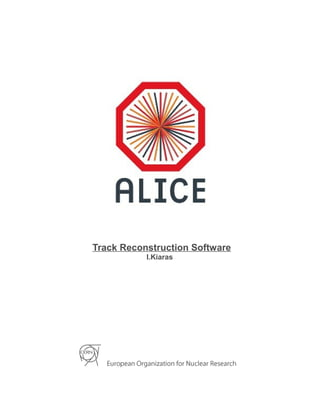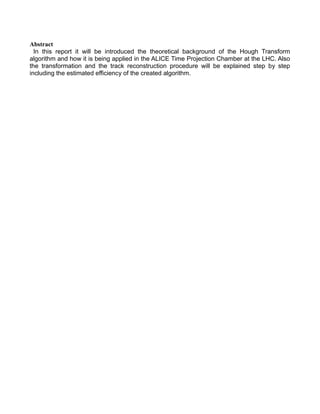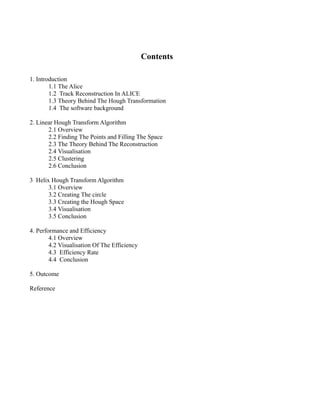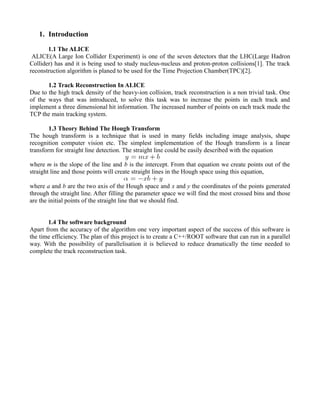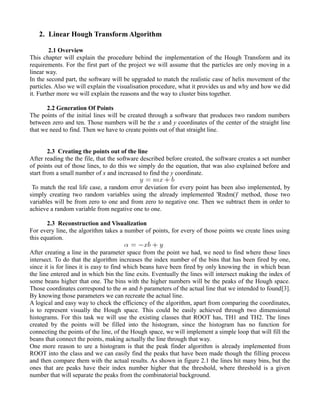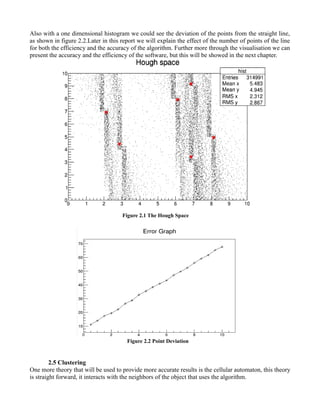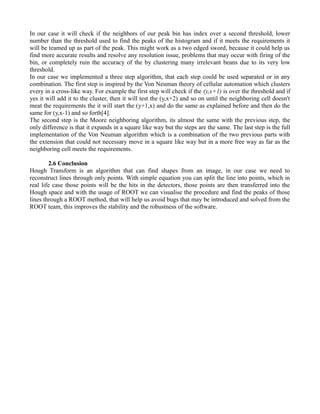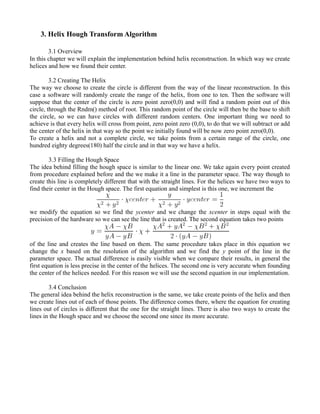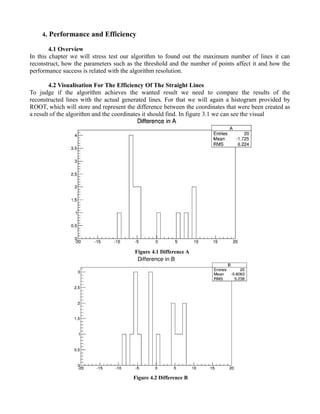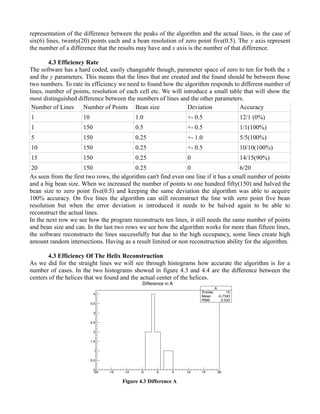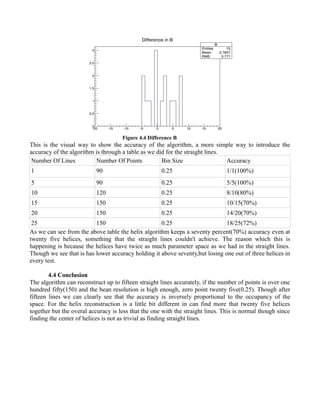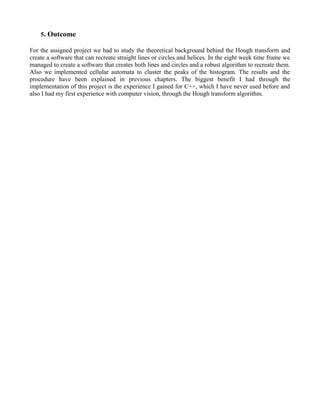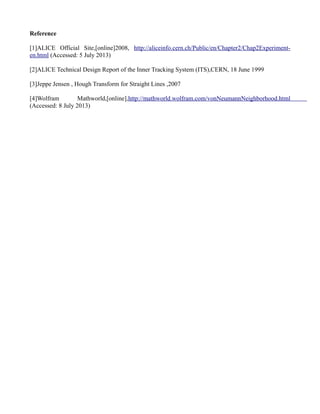Próximo SlideShare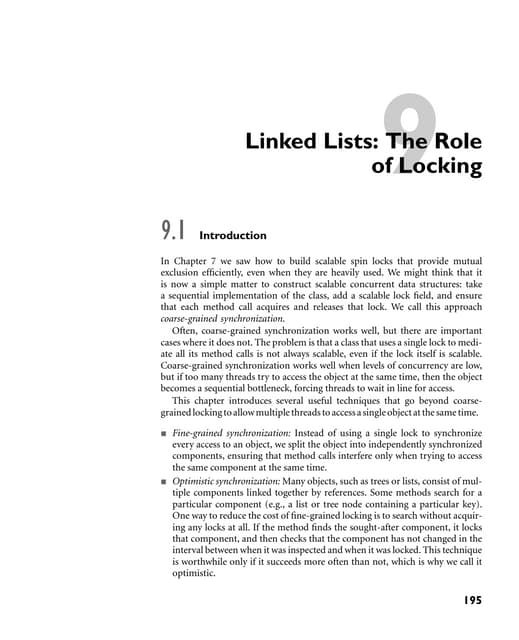Linked Lists: The Role of Locking : The Art of Multiprocessor Programming : N...
Cargando en ... 3
1 de 13

### Kiaras Ioannis cern

1. Track Reconstruction Software I.Kiaras
2. Abstract In this report it will be introduced the theoretical background of the Hough Transform algorithm and how it is being applied in the ALICE Time Projection Chamber at the LHC. Also the transformation and the track reconstruction procedure will be explained step by step including the estimated efficiency of the created algorithm.
3. Contents 1. Introduction 1.1 The Alice 1.2 Track Reconstruction In ALICE 1.3 Theory Behind The Hough Transformation 1.4 The software background 2. Linear Hough Transform Algorithm 2.1 Overview 2.2 Finding The Points and Filling The Space 2.3 The Theory Behind The Reconstruction 2.4 Visualisation 2.5 Clustering 2.6 Conclusion 3 Helix Hough Transform Algorithm 3.1 Overview 3.2 Creating The circle 3.3 Creating the Hough Space 3.4 Visualisation 3.5 Conclusion 4. Performance and Efficiency 4.1 Overview 4.2 Visualisation Of The Efficiency 4.3 Efficiency Rate 4.4 Conclusion 5. Outcome Reference
4. 1. Introduction 1.1 The ALICE ALICE(A Large Ion Collider Experiment) is one of the seven detectors that the LHC(Large Hadron Collider) has and it is being used to study nucleus-nucleus and proton-proton collisions. The track reconstruction algorithm is planed to be used for the Time Projection Chamber(TPC). 1.2 Track Reconstruction In ALICE Due to the high track density of the heavy-ion collision, track reconstruction is a non trivial task. One of the ways that was introduced, to solve this task was to increase the points in each track and implement a three dimensional hit information. The increased number of points on each track made the TCP the main tracking system. 1.3 Theory Behind The Hough Transform The hough transform is a technique that is used in many fields including image analysis, shape recognition computer vision etc. The simplest implementation of the Hough transform is a linear transform for straight line detection. The straight line could be easily described with the equation where m is the slope of the line and b is the intercept. From that equation we create points out of the straight line and those points will create straight lines in the Hough space using this equation, where a and b are the two axis of the Hough space and x and y the coordinates of the points generated through the straight line. After filling the parameter space we will find the most crossed bins and those are the initial points of the straight line that we should find. 1.4 The software background Apart from the accuracy of the algorithm one very important aspect of the success of this software is the time efficiency. The plan of this project is to create a C++/ROOT software that can run in a parallel way. With the possibility of parallelisation it is believed to reduce dramatically the time needed to complete the track reconstruction task.
5. 2. Linear Hough Transform Algorithm 2.1 Overview This chapter will explain the procedure behind the implementation of the Hough Transform and its requirements. For the first part of the project we will assume that the particles are only moving in a linear way. In the second part, the software will be upgraded to match the realistic case of helix movement of the particles. Also we will explain the visualisation procedure, what it provides us and why and how we did it. Further more we will explain the reasons and the way to cluster bins together. 2.2 Generation Of Points The points of the initial lines will be created through a software that produces two random numbers between zero and ten. Those numbers will be the x and y coordinates of the center of the straight line that we need to find. Then we have to create points out of that straight line. 2.3 Creating the points out of the line After reading the the file, that the software described before created, the software creates a set number of points out of those lines, to do this we simply do the equation, that was also explained before and start from a small number of x and increased to find the y coordinate. To match the real life case, a random error deviation for every point has been also implemented, by simply creating two random variables using the already implemented 'Rndm()' method, those two variables will be from zero to one and from zero to negative one. Then we subtract them in order to achieve a random variable from negative one to one. 2.3 Reconstruction and Visualization For every line, the algorithm takes a number of points, for every of those points we create lines using this equation. After creating a line in the parameter space from the point we had, we need to find where those lines intersect. To do that the algorithm increases the index number of the bins that has been fired by one, since it is for lines it is easy to find which beans have been fired by only knowing the in which bean the line entered and in which bin the line exits. Eventually the lines will intersect making the index of some beans higher that one. The bins with the higher numbers will be the peaks of the Hough space. Those coordinates correspond to the m and b parameters of the actual line that we intended to found. By knowing those parameters we can recreate the actual line. A logical and easy way to check the efficiency of the algorithm, apart from comparing the coordinates, is to represent visually the Hough space. This could be easily achieved through two dimensional histograms. For this task we will use the existing classes that ROOT has, TH1 and TH2. The lines created by the points will be filled into the histogram, since the histogram has no function for connecting the points of the line, of the Hough space, we will implement a simple loop that will fill the beans that connect the points, making actually the line through that way. One more reason to ure a histogram is that the peak finder algorithm is already implemented from ROOT into the class and we can easily find the peaks that have been made though the filling process and then compare them with the actual results. As shown in figure 2.1 the lines hit many bins, but the ones that are peaks have their index number higher that the threshold, where threshold is a given number that will separate the peaks from the combinatorial background.
6. Also with a one dimensional histogram we could see the deviation of the points from the straight line, as shown in figure 2.2.Later in this report we will explain the effect of the number of points of the line for both the efficiency and the accuracy of the algorithm. Further more through the visualisation we can present the accuracy and the efficiency of the software, but this will be showed in the next chapter. Figure 2.1 The Hough Space Figure 2.2 Point Deviation 2.5 Clustering One more theory that will be used to provide more accurate results is the cellular automaton, this theory is straight forward, it interacts with the neighbors of the object that uses the algorithm.
7. In our case it will check if the neighbors of our peak bin has index over a second threshold, lower number than the threshold used to find the peaks of the histogram and if it meets the requirements it will be teamed up as part of the peak. This might work as a two edged sword, because it could help us find more accurate results and resolve any resolution issue, problems that may occur with firing of the bin, or completely ruin the accuracy of the by clustering many irrelevant beans due to its very low threshold. In our case we implemented a three step algorithm, that each step could be used separated or in any combination. The first step is inspired by the Von Neuman theory of cellular automation which clusters every in a cross-like way. For example the first step will check if the (y,x+1) is over the threshold and if yes it will add it to the cluster, then it will test the (y,x+2) and so on until the neighboring cell doesn't meat the requirements the it will start the (y+1,x) and do the same as explained before and then do the same for (y,x-1) and so forth. The second step is the Moore neighboring algorithm, its almost the same with the previous step, the only difference is that it expands in a square like way but the steps are the same. The last step is the full implementation of the Von Neuman algorithm which is a combination of the two previous parts with the extension that could not necessary move in a square like way but in a more free way as far as the neighboring cell meets the requirements. 2.6 Conclusion Hough Transform is an algorithm that can find shapes from an image, in our case we need to reconstruct lines through only points. With simple equation you can split the line into points, which in real life case those points will be the hits in the detectors, those points are then transferred into the Hough space and with the usage of ROOT we can visualise the procedure and find the peaks of those lines through a ROOT method, that will help us avoid bugs that may be introduced and solved from the ROOT team, this improves the stability and the robustness of the software.
8. 3. Helix Hough Transform Algorithm 3.1 Overview In this chapter we will explain the implementation behind helix reconstruction. In which way we create helices and how we found their center. 3.2 Creating The Helix The way we choose to create the circle is different from the way of the linear reconstruction. In this case a software will randomly create the range of the helix, from one to ten. Then the software will suppose that the center of the circle is zero point zero(0,0) and will find a random point out of this circle, through the Rndm() method of root. This random point of the circle will then be the base to shift the circle, so we can have circles with different random centers. One important thing we need to achieve is that every helix will cross from point, zero point zero (0,0), to do that we will subtract or add the center of the helix in that way so the point we initially found will be now zero point zero(0,0). To create a helix and not a complete circle, we take points from a certain range of the circle, one hundred eighty degrees(180) half the circle and in that way we have a helix. 3.3 Filling the Hough Space The idea behind filling the hough space is similar to the linear one. We take again every point created from procedure explained before and the we make it a line in the parameter space. The way though to create this line is completely different that with the straight lines. For the helices we have two ways to find their center in the Hough space. The first equation and simplest is this one, we increment the we modify the equation so we find the ycenter and we change the xcenter in steps equal with the precision of the hardware so we can see the line that is created. The second equation takes two points of the line and creates the line based on them. The same procedure takes place in this equation we change the x based on the resolution of the algorithm and we find the y point of the line in the parameter space. The actual difference is easily visible when we compare their results, in general the first equation is less precise in the center of the helices. The second one is very accurate when founding the center of the helices needed. For this reason we will use the second equation in our implementation. 3.4 Conclusion The general idea behind the helix reconstruction is the same, we take create points of the helix and then we create lines out of each of those points. The difference comes there, where the equation for creating lines out of circles is different that the one for the straight lines. There is also two ways to create the lines in the Hough space and we choose the second one since its more accurate.
9. 4. Performance and Efficiency 4.1 Overview In this chapter we will stress test our algorithm to found out the maximum number of lines it can reconstruct, how the parameters such as the threshold and the number of points affect it and how the performance success is related with the algorithm resolution. 4.2 Visualisation For The Efficiency Of The Straight Lines To judge if the algorithm achieves the wanted result we need to compare the results of the reconstructed lines with the actual generated lines. For that we will again a histogram provided by ROOT, which will store and represent the difference between the coordinates that were been created as a result of the algorithm and the coordinates it should find. In figure 3.1 we can see the visual Figure 4.1 Difference A Figure 4.2 Difference B
10. representation of the difference between the peaks of the algorithm and the actual lines, in the case of six(6) lines, twenty(20) points each and a bean resolution of zero point five(0.5). The y axis represent the number of a difference that the results may have and x axis is the number of that difference. 4.3 Efficiency Rate The software has a hard coded, easily changeable though, parameter space of zero to ten for both the x and the y parameters. This means that the lines that are created and the found should be between those two numbers. To rate its efficiency we need to found how the algorithm responds to different number of lines, number of points, resolution of each cell etc. We will introduce a small table that will show the most distinguished difference between the numbers of lines and the other parameters. Number of Lines Number of Points Bean size Deviation Accuracy 1 10 1.0 +- 0.5 12/1 (0%) 1 150 0.5 +- 0.5 1/1(100%) 5 150 0.25 +- 1.0 5/5(100%) 10 150 0.25 +- 0.5 10/10(100%) 15 150 0.25 0 14/15(90%) 20 150 0.25 0 6/20 As seen from the first two rows, the algorithm can't find even one line if it has a small number of points and a big bean size. When we increased the number of points to one hundred fifty(150) and halved the bean size to zero point five(0.5) and keeping the same deviation the algorithm was able to acquire 100% accuracy. On five lines the algorithm can still reconstruct the line with zero point five bean resolution but when the error deviation is introduced it needs to be halved again to be able to reconstruct the actual lines. In the next row we see how the program reconstructs ten lines, it still needs the same number of points and bean size and can. In the last two rows we see how the algorithm works for more than fifteen lines, the software reconstructs the lines successfully but due to the high occupancy, some lines create high amount random intersections. Having as a result limited or non reconstruction ability for the algorithm. 4.3 Efficiency Of The Helix Reconstruction As we did for the straight lines we will see through histograms how accurate the algorithm is for a number of cases. In the two histograms showed in figure 4.3 and 4.4 are the difference between the centers of the helices that we found and the actual center of the helices. Figure 4.3 Difference A
11. Figure 4.4 Difference B This is the visual way to show the accuracy of the algorithm, a more simple way to introduce the accuracy of the algorithm is through a table as we did for the straight lines. Number Of Lines Number Of Points Bin Size Accuracy 1 90 0.25 1/1(100%) 5 90 0.25 5/5(100%) 10 120 0.25 8/10(80%) 15 150 0.25 10/15(70%) 20 150 0.25 14/20(70%) 25 150 0.25 18/25(72%) As we can see from the above table the helix algorithm keeps a seventy percent(70%) accuracy even at twenty five helices, something that the straight lines couldn't achieve. The reason which this is happening is because the helices have twice as much parameter space as we had in the straight lines. Though we see that is has lower accuracy holding it above seventy,but losing one out of three helices in every test. 4.4 Conclusion The algorithm can reconstruct up to fifteen straight lines accurately, if the number of points is over one hundred fifty(150) and the bean resolution is high enough, zero point twenty five(0.25). Though after fifteen lines we can clearly see that the accuracy is inversely proportional to the occupancy of the space. For the helix reconstruction is a little bit different in can find more that twenty five helices together but the overal accuracy is less that the one with the straight lines. This is normal though since finding the center of helices is not as trivial as finding straight lines.
12. 5. Outcome For the assigned project we had to study the theoretical background behind the Hough transform and create a software that can recreate straight lines or circles and helices. In the eight week time frame we managed to create a software that creates both lines and circles and a robust algorithm to recreate them. Also we implemented cellular automata to cluster the peaks of the histogram. The results and the procedure have been explained in previous chapters. The biggest benefit I had through the implementation of this project is the experience I gained for C++, which I have never used before and also I had my first experience with computer vision, through the Hough transform algorithm.
13. Reference ALICE Official Site,[online]2008, http://aliceinfo.cern.ch/Public/en/Chapter2/Chap2Experiment- en.html (Accessed: 5 July 2013) ALICE Technical Design Report of the Inner Tracking System (ITS),CERN, 18 June 1999 Jeppe Jensen , Hough Transform for Straight Lines ,2007 Wolfram Mathworld,[online],http://mathworld.wolfram.com/vonNeumannNeighborhood.html (Accessed: 8 July 2013)